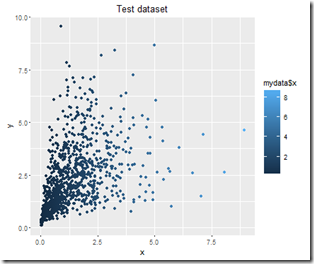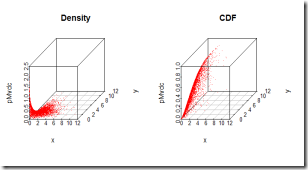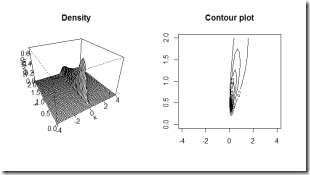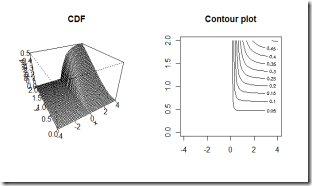Want to share your content on R-bloggers? click here if you have a blog, or here if you don't.

Here I am again with part 2. If you would like to read part 1 of this short tutorial on copulas, please click here.

In this second post I am going to select a copula model, fit it to a test dataset, evaluate the fitting and generate random observations from the fitted multivariate distribution. Furthermore I am going to show how to measure correlation using Spearman’s Rho and Kendall’s Tau. In order to run the code in this post you need the following packages: copula and VineCopula.

## The dataset

For this example I am going to use a test dataset. You can download it from this link. This test dataset contains two variables, x and y, characterized by a strong left tail correlation.

By visually inspecting the plot of x versus y you can easily notice that low values of x tends to be highly correlated with low values of y.## The x and y distributions

First of all, let’s study the marginals separately. Coming up with an estimate of the marginal distributions for both x and y should not be that hard. In order to do this, a quick check to the histogram of both variables could provide some insights.

A good glimpse at the chart of each variable is important in order to get a grasp of what distribution could be a better fit. It looks like a Gamma distribution could do both for x and y. This is just a quick guess, obviously it would be necessary to dig deeper and inspect the data further before making such a claim, however this is not the focus of this post. Moving on pretty fast and assuming the distribution assumption is correct, the only thing left is to estimate the parameters. Let’s estimate the parameters, sample some observations from the fitted distributions and make a visual comparison.

You can see in green the observed data and in blue the simulated data for both x and y. That looks like a good match.

## Measures of association Kendall tau, Spearman’s rho

Now it’s time to look at the joint behaviour. For instance, we could measure the association between x and y. When dealing with copulas, two common measures of association are Kendall’s Tau and Spearman’s Rho. These two are usually better suited for measuring association than linear correlation measures when working with copulas. I am going to use Kendall’s Tau

Take note of the output of this code because later, when you will have fit the copula model, you will be able to check that the same correlation structure has been preserved (or captured, if you wish) by the copula.

## Selecting the copula using VineCopula package

Since we are dealing with a bivariate case, we can use a scatterplot to get an idea of what the joynt relationship of x and y looks like and try to understand the joint behaviour of the two variables. As you know, the joint behaviour is captured by the copula, therefore by taking a look at it we can try to guess what copula might fit.

Of course a guess is not the optimal way of selecting a model, furthermore the “visual guessing method” is not applicable in case we have more than 3 variables, so we are going to use a useful function provided by the Vinecopula package.

The VineCopula package provides a convenient way of choosing a copula through the BiCopSelect() function. The VineCopula library performs copula selection using BIC and AIC.

Note that BiCopSelect() accepts pseudo observations as parameters. That is, the fitting process is performed on the pseudo observations, ie the observations in the unit square \$[0,1]^2\$. The pobs() function takes care of the conversion from real observations to pseudo observations. Note that pobs() returns a matrix (not a dataframe!).

It appears that a Clayton copula might be a good choice for our problem. The unique parameter of the Clayton, theta, is estimated to be 1.48.

## Fitting process with a given copula

The BiCopSelect function, estimates the copula parameters too. However, in case you already know what copula to use, you could fit it using the fitCopula() function.

It looks like the fitting process was successful and the parameters of the fitted copula are the same estimated with the BiCopSelect() function. That’s a good double check, by the way. Note that Kendall’s tau is the same as the one calculated from the observed x and y.

## Goodness of fit test

Once the copula has been fitted, we can test to check whether the fit is good or not. In order to perform this GOF test, we can use the gofCopula() function as below. Note that this test may be a little slow to run.
For comparison, I decided to run it twice, first with a normal copula and then with a Clayton.

## Building the bivariate distribution using the copula

Now that we have successfully chosen and fitted the copula to the data and selected the appropriate marginals, we can try to model the joint relationship using the basic tools I showed in part 1 of this short tutorial.Now we can sample some observations from the estimated joint distribution and check how they compare to the one observed.

## Sample observations from the brand new bivariate distribution

Simply use the tools I explained in part 1 to sample from the new built distribution.Note that Kendall’s tau is still the same for the simulated data. The information about the correlation structure has been captured by the copula regardless of the marginals. Of course this is just a taste of how powerful plain vanilla copulas, such as Archimedean copulas, can be.

At the top of my mind I cannot think of other “easy to use” tools for modelling “weird” relationships such as the one between x and y in my test dataset.

I hope this two parts post was useful and interesting. Please feel free to leave a comment if you have any question or spot any mistake.